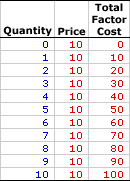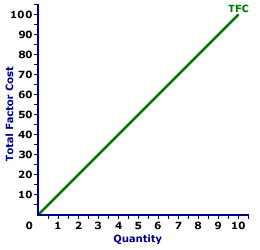Saturday  June 25, 2022
 AmosWEB means Economics with a Touch of Whimsy!AGGREGATE EXPENDITURE DETERMINANT: A ceteris paribus factor that affects aggregate expenditures, but which is assumed constant when the aggregate expenditure line is constructed. Changes in any of the aggregate expenditures determinants cause the aggregate expenditure line to shift. While a wide variety of specific ceteris paribus factors can cause the aggregate expenditure line to shift, it's usually most convenient to group them into the four, broad expenditure categories -- consumption, investment, government purchases, and net exports. The reason is that changes in these expenditures are the direct cause of shifts in the aggregate expenditure line. If any determinant affects aggregate expenditures it MUST affect one of these four expenditures.TOTAL FACTOR COST, PERFECT COMPETITION:

The opportunity cost incurred by a perfectly competitive firm when using a given factor of production to produce a good or service. This is the total cost associated with the use of a particular resource or factor of production--it is the total cost of the factor. For a perfectly competitive firm, the price paid is constant and total factor cost increases at a constant rate. Total factor cost is predominately used in the analysis of the factor market. Two derivative factor cost measures are average factor cost and marginal factor cost.
Total factor cost is the total opportunity cost incurred by a perfectly competitive firm from the employment of a given resource. This measure of cost needs to be contrasted with a similar, better known term, total cost. Total cost is the cost of all factors of production, whereas total factor cost is the cost of using a specific factor. Total cost, as such, is the sum of the total factor cost of labor, the total factor cost of capital, and the total factor cost of all other inputs.

Total factor cost can be represented in a table or as a curve. For a perfectly competitive firm with no market control hiring inputs under perfect competition, the total factor cost curve is a straight line that emerges from the origin. For firms with market control, including monopsony, oligopsony, or monopsonistic competition, the total factor cost curve increases at an increasing rate. The shape of the total factor cost curve thus indicates the degree of market control possessed by the factor buyer.

Whichever market structure is involved, total factor cost is calculated as the factor price times the quantity of the factor purchased, as illustrated by this equation:

 total factor cost = factor price x factor quantity
If the firm is hiring the factor in a perfectly competitive factor market, then the factor price is fixed or constant and total factor cost increases at a constant rate. If the firm is hiring the factor in an imperfectly competitive factor market, best illustrated by monopsony, then the factor price increases with larger factor quantities and the total factor cost increases at an increasing rate.

The basics of total factor cost can be illustrated by perfect competition. Perfect competition is a market structure with a large number of small participants (buyers and sellers). The good exchanged in the market is identical, regardless of who sells or who buys. Participants have perfect knowledge and perfect mobility into and out of the market. These conditions mean perfectly competitive buyers are price takers, they have no market control and must pay the going market price for all inputs bought.

Total Factor Cost,
Perfect CompetitionThe table to the right summarizes the total factor cost incurred by a hypothetical buyer, Maggie's Macrame Shoppe, for hiring store clerks in a perfectly competitive labor market. Maggie's Macrame Shoppe is one of thousands of small retail stores in the greater Shady Valley metropolitan area that hires labor with identical skills. As such, Maggie pays the going wage for labor.

The first column in the table is the quantity of workers hired, ranging from 0 to 10 workers. The second column is the price Maggie pays for hiring her workers, which is constant at \$10 per worker. The third column is then the total factor cost Maggie incurs for hiring various numbers of workers. If Maggie hires only one worker, then she pays only \$10. If she hires five workers, she pays \$50. In each case, total factor cost in column three is calculated as the quantity in the first column multiplied by the price in the second column.

Consider a few highlights of these total factor cost numbers.

• First, total factor cost is zero if Maggie hires no workers. This makes sense. If nothing is bought, no cost is incurred.

• Second, the price remains unchanged. Maggie can hire 1 worker or 10 workers for the same \$10 wage. Presumably, if Maggie hired 100 workers, the wage remains at \$10.

• Third, as Maggie hires more workers she incurs more total factor cost.

• Fourth and last, for each extra worker Maggie hires, she pays an extra \$10 of cost. This suggests an important role for marginal factor cost in the factor market analysis of a perfectly competitive firm.
Total Factor Cost Curve,
Perfect CompetitionTotal factor cost is commonly represented by a total factor cost curve, such as the one displayed in the exhibit to the right. This particular total factor cost curve is that for labor hired by Maggie's Macrame Shoppe.

The vertical axis measures total factor cost and the horizontal axis measures the quantity of input (workers). Although quantity on this particular graph stops at 10 workers, the nature of perfect competition indicates it could go higher.

This curve indicates that if Maggie hires 1 worker, then she pays \$10 of total factor cost. Alternatively, if she hires 10 workers, then she pays \$100 of total factor cost. Should she hire 100 workers, then she would move well beyond the graph, with \$1000 of total factor cost.

The "curve" is actually a "straight line" because Maggie is a price taker in the labor market. She pays \$10 for each worker whether she hires 1 worker or 10 workers. The constant price is what makes Maggie's total factor cost curve a straight line, and which indicates that Maggie has no market control.

 <= TOTAL FACTOR COST, MONOPSONY TOTAL FIXED COST =>Recommended Citation:

TOTAL FACTOR COST, PERFECT COMPETITION, AmosWEB Encyclonomic WEB*pedia, http://www.AmosWEB.com, AmosWEB LLC, 2000-2022. [Accessed: June 25, 2022].

Check Out These Related Terms...

Or For A Little Background...

And For Further Study...
Search Again?BEIGE MUNDORTLE[What's This?] Today, you are likely to spend a great deal of time calling an endless list of 800 numbers wanting to buy either a large green chalkboard shaped like the state of Maine or a replacement battery for your pocket calculator. Be on the lookout for attractive cable television service repair people.Your Complete ScopeOne of the largest markets for gold in the United States is the manufacturing of class rings."It is not fair to ask of others what you are unwilling to do yourself. "-- Eleanor Roosevelt, diplomat, activistVATValue Added TaxA PEDestrian's Guide Xtra CreditTell us what you think about AmosWEB. Like what you see? Have suggestions for improvements? Let us know. Click the User Feedback link.| | | | | | | | | | |
| | | |

Thanks for visiting AmosWEB